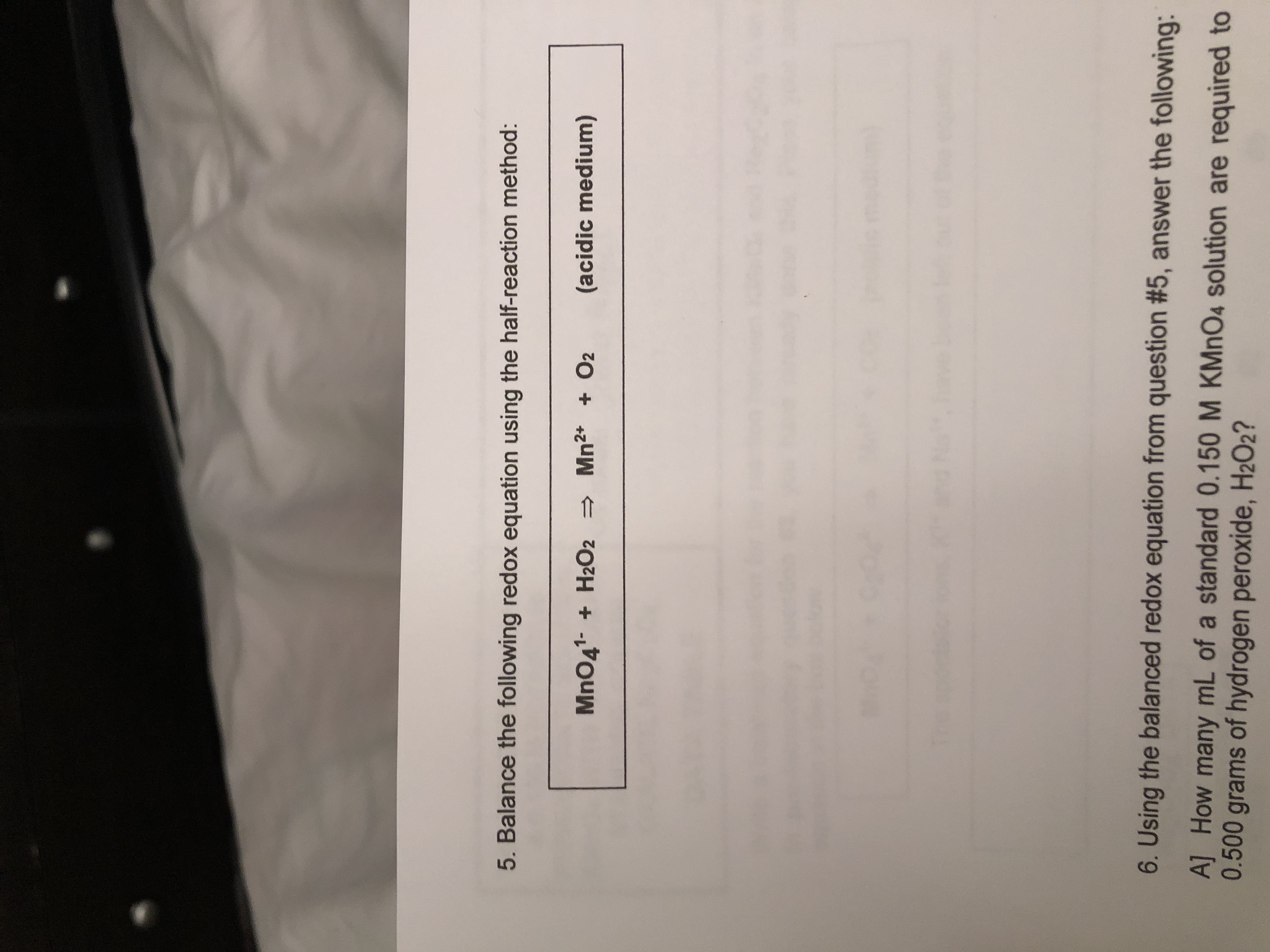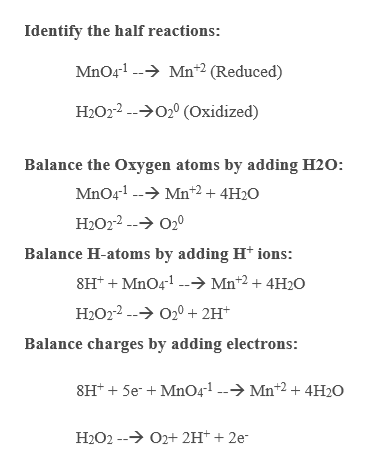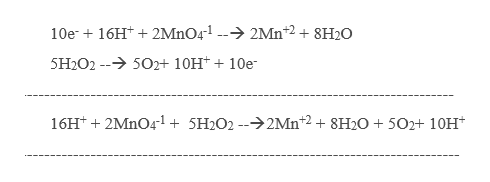# 5. Balance the following redox equation using the half-reaction method:MnO41-H2O2 Mn2 O2(acidic medium)6. Using the balanced redox equation from question #5, answer the following:A] How many mL of a standard 0.150 M KMnO4 solution are required to0.500 grams of hydrogen peroxide, H2O2?

Question

Question 5help_outlineImage Transcriptionclose5. Balance the following redox equation using the half-reaction method: MnO41-H2O2 Mn2 O2 (acidic medium) 6. Using the balanced redox equation from question #5, answer the following: A] How many mL of a standard 0.150 M KMnO4 solution are required to 0.500 grams of hydrogen peroxide, H2O2? fullscreen
check_circle

Step 1

The given redox reaction in acidic medium can be Balanced by step by step as shown below,help_outlineImage TranscriptioncloseIdentify the half reactions: MnO41 Mn2 (Reduced) H2O22 (Oxidized) Balance the Oxygen atoms by adding H20 MnO41 Mn2 + 4H2O H2O22 O20 Balance H-atoms by adding H ions: 8HMnO4 Mn*2 + 4H20 H2O2 202H* Balance charges by adding electrons: 8H*5eMnO41 --->Mnt2 + 4H20 O2+ 2H+ + 2e H2O2- fullscreen
Step 2

Multiply the above two equations with 2 and 5 re...help_outlineImage Transcriptionclose10e16H2MnO4-1 -2Mn2 +8H20 5H2O2 -502+ 10H10e 16Ht2MnO41 + 5H2O2 --2Mn 2 + 8H20 +502+ 10H* fullscreen

### Want to see the full answer?

See Solution

#### Want to see this answer and more?

Solutions are written by subject experts who are available 24/7. Questions are typically answered within 1 hour.*

See Solution
*Response times may vary by subject and question.
Tagged in

### Redox Reactions